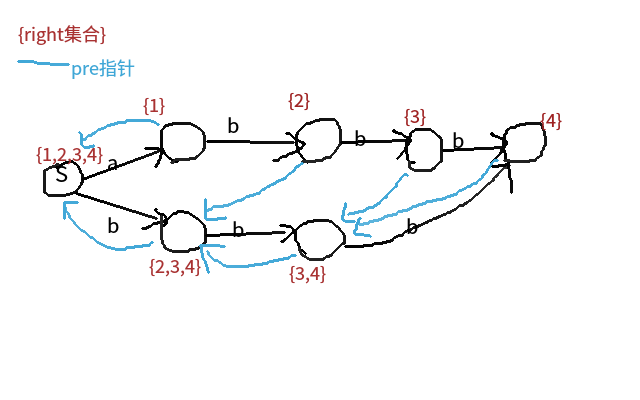# What is 后缀自动机？# 后缀自动机的一些相关定义

step(x)： 表示从起始点出发，最多走几步可以走到节点 x，也就是节点 x 可以代表的最长子串的长度。有些资料里将其称为 len

pre 指针：与 AC 自动机的 fail 指针类似，是失配指针。pre(x) 可以代表的子串，x 一定可以代表，并且 pre(x) 可以代表的子串，是 x 可以代表的子串的长度连续后缀，而且 pre(x) 可以代表的最长子串，是 x 可以代表的最短子串的长度减 1。

right 集合：又名 end-pos 集合（咋感觉后缀自动机的命名很不统一啊）。顾名思义，假设 $t \in right(x)$。x 节点可以代表的子串，是原串中以第 t 个字符结尾的前缀的后缀。

# 如何构建后缀自动机

int np=++SZ,p=last; last=np,step[np]=step[p]+1;
while(!ch[p][x]&&p) ch[p][x]=np,p=pre[p];


//初始 last=SZ=1
void ins(int x) {
int np=++SZ,p=last; last=np,step[np]=step[p]+1;
while(!ch[p][x]&&p) ch[p][x]=np,p=pre[p];
if(!p) pre[np]=1;
else {
int q=ch[p][x];
if(step[q]==step[p]+1) pre[np]=q;
else {
int nq=++SZ;step[nq]=step[p]+1;
for(RI i=0;i<26;++i) ch[nq][i]=ch[q][i];
pre[nq]=pre[q],pre[q]=pre[np]=nq;
while(ch[p][x]==q&&p) ch[p][x]=nq,p=pre[p];
}
}
}


# 一些例题

## 例 1

bzoj3998

#include<bits/stdc++.h>
using namespace std;
#define RI register int
const int N=1000005;
char S[N];int ch[N],step[N],pre[N],T[N],a[N];
int sz[N],sum[N];
int ty,K,SZ,last,n;

void ins(int x) {
int np=++SZ,p=last;
last=np,step[np]=step[p]+1,sz[np]=1;
while(!ch[p][x]&&p) ch[p][x]=np,p=pre[p];
if(!p) pre[np]=1;
else {
int q=ch[p][x];
if(step[q]==step[p]+1) pre[np]=q;
else {
int nq=++SZ;step[nq]=step[p]+1;
for(RI i=0;i<26;++i) ch[nq][i]=ch[q][i];
pre[nq]=pre[q],pre[q]=pre[np]=nq;
while(ch[p][x]==q&&p) ch[p][x]=nq,p=pre[p];
}
}
}
void prework() {
for(RI i=1;i<=SZ;++i) ++T[step[i]];//利用后缀自动机性质拓扑排序
for(RI i=1;i<=SZ;++i) T[i]+=T[i-1];
for(RI i=1;i<=SZ;++i) a[T[step[i]]--]=i;
for(RI i=SZ;i>=1;--i)
if(ty) sz[pre[a[i]]]+=sz[a[i]];
else sz[i]=1;
sz=0;
for(RI i=SZ;i>=1;--i) {
int x=a[i];sum[x]=sz[x];//sum: 停下或继续，你还能走出多少个子串
for(RI j=0;j<26;++j) if(ch[x][j]) sum[x]+=sum[ch[x][j]];
}
}
void work() {
if(sum<K) {puts("-1");return;}
int now=1;
while(1) {
if(K<=sz[now]) break;
K-=sz[now];
for(RI i=0;i<26;++i)
if(sum[ch[now][i]]<K) K-=sum[ch[now][i]];
else {now=ch[now][i],printf("%c",i+'a');break;}
}
}
int main()
{
scanf("%s",S+1),n=strlen(S+1);
scanf("%d%d",&ty,&K);
last=SZ=1;for(RI i=1;i<=n;++i) ins(S[i]-'a');
prework(),work();
return 0;
}


## 例 2

bzoj3238

DP 即可。

#include<bits/stdc++.h>
using namespace std;
#define RI register int
typedef long long LL;
const int N=1000005;
char S[N];int ch[N],pre[N],step[N],T[N],p[N],sz[N];
int n,SZ,last,tot;LL ans;

void ins(int x) {
int np=++SZ,p=last;
last=np,step[np]=step[p]+1,sz[np]=1;
while(!ch[p][x]&&p) ch[p][x]=np,p=pre[p];
if(!p) pre[np]=1;
else {
int q=ch[p][x];
if(step[q]==step[p]+1) pre[np]=q;
else {
int nq=++SZ;step[nq]=step[p]+1;
for(RI i=0;i<26;++i) ch[nq][i]=ch[q][i];
pre[nq]=pre[q],pre[q]=pre[np]=nq;
while(ch[p][x]==q&&p) ch[p][x]=nq,p=pre[p];
}
}
}
void DP() {
for(RI i=1;i<=SZ;++i) ++T[step[i]];
for(RI i=1;i<=SZ;++i) T[i]+=T[i-1];
for(RI i=1;i<=SZ;++i) p[T[step[i]]--]=i;
for(RI i=SZ;i>=1;--i) {
int x=p[i];
ans+=1LL*sz[pre[x]]*sz[x]*step[pre[x]],sz[pre[x]]+=sz[x];
}
}
int main()
{
scanf("%s",S+1),n=strlen(S+1);
last=SZ=1;for(RI i=n;i>=1;--i) ins(S[i]-'a');
DP();ans=1LL*(n-1)*n*(n+1)/2-ans*2;
printf("%lld\n",ans);
return 0;
}


## 例 3

bzoj3277

set 启发式合并处理每个节点代表了哪些字符串的子串，set 集合的大小大于 K 的那些节点代表的子串就是符合条件的，就把这些节点的权值设为它代表的字符串个数（step(x)-step(pre(x))），否则设为 0。

#include<bits/stdc++.h>
using namespace std;
#define RI register int
typedef long long LL;
const int N=200005;
char S[N];
int ch[N],pre[N],step[N],id[N];
int h[N],ne[N],to[N];LL val[N],ans[N];
set<int> orz[N];
int n,K,SZ,last,tot;

void ins(int ww,int x) {
int np=++SZ,p=last;
last=np,step[np]=step[p]+1,id[np]=ww,orz[np].insert(ww);
while(!ch[p][x]&&p) ch[p][x]=np,p=pre[p];
if(!p) pre[np]=1;
else {
int q=ch[p][x];
if(step[q]==step[p]+1) pre[np]=q;
else {
int nq=++SZ;step[nq]=step[p]+1;
for(RI i=0;i<26;++i) ch[nq][i]=ch[q][i];
pre[nq]=pre[q],pre[q]=pre[np]=nq;
while(ch[p][x]==q&&p) ch[p][x]=nq,p=pre[p];
}
}
}

typedef set<int>::iterator itr;
void add(int x,int y) {to[++tot]=y,ne[tot]=h[x],h[x]=tot;}
void dfs1(int x) {
for(RI i=h[x];i;i=ne[i]) {
dfs1(to[i]);
if((int)orz[to[i]].size()>(int)orz[x].size()) swap(orz[to[i]],orz[x]);
for(itr j=orz[to[i]].begin();j!=orz[to[i]].end();++j) orz[x].insert(*j);
orz[to[i]].clear();
}
if((int)orz[x].size()>=K) val[x]=step[x]-step[pre[x]];
}
void dfs2(int x,LL nowval) {
nowval+=val[x];
if(id[x]) ans[id[x]]+=nowval;
for(RI i=h[x];i;i=ne[i]) dfs2(to[i],nowval);
}
int main()
{
scanf("%d%d",&n,&K);
SZ=1;
for(RI i=1;i<=n;++i) {
scanf("%s",S+1);
int len=strlen(S+1);last=1;
for(RI j=1;j<=len;++j) ins(i,S[j]-'a');
}
dfs1(1),dfs2(1,0);
for(RI i=1;i<=n;++i) printf("%lld ",ans[i]);
return 0;
}


# 题外话：子序列自动机

void ins(int x) {
++SZ,pre[SZ]=last[x];
for(RI i=0;i<26;++i) {
int now=last[i];
while(!ch[now][x]) ch[now][x]=SZ,now=pre[now];
}
last[x]=SZ;
}


### 4 条评论#### yyb · 2018年2月26日 7:41 下午Orz 太强了，都学后缀自动机了